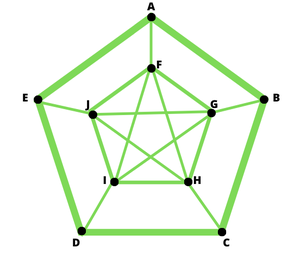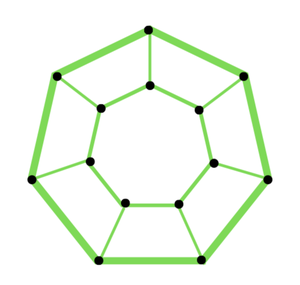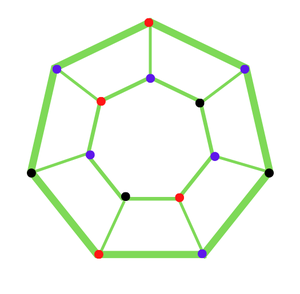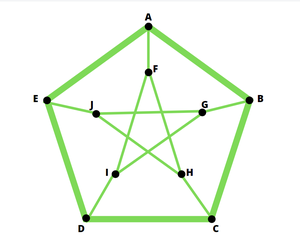Open in App
Not now

# What is Peterson Graph?

• Difficulty Level : Medium
• Last Updated : 12 Sep, 2022

A graph is a data structure that is defined by two components :

• A node or a vertex.
• An edge E or ordered pair is a connection between two nodes u,v that is identified by unique pair(u,v). The pair (u,v) is ordered because (u,v) is not the same as (v,u) in the case of a directed graph. The edge may have a weight or is set to one in case of an unweighted graph.

### Peterson Graph:

The Peterson graph

• is a cubic graph with 10 vertices and 15 edges.
• is a unique (3,5)-cage graph and the unique (3,5)-Moore graph.
• is the odd graph with parameter 3. This is the Kneser graph wherein two vertices are adjacent if and only if the corresponding subsets are disjoint.
• is also a complement of the line graph k
• is the smallest bridgeless 3-regular graph with no edge 3-coloring
• has the most spanning trees of any 3-regular graph with ten vertices, with 2000.

There is no 3-cycle or 4-cycle in the Peterson Graph.Peterson graph

Construction

The Peterson graph is made up of the vertices and edges of a Hemi-dodecahedron, which is a dodecahedron with opposite points, lines, and faces identified together.

### Generalized Peterson graphs

A family of cubic graphs produced by connecting the vertices of a regular polygon to the equivalent vertices of a star polygon is known as a generalized Peterson graph. The generalized Peterson graphs are denoted by P(n,k).P(7,1)

If n=7 , k = 7/2 =3 , P(7,1); P(7,2); P(7,3)

### Chromatic number of Peterson Graph:

The Graph, as shown in the above figure, is not complete. Furthermore, it has an odd number of edges. As a result, it is not a bipartite graph.

As the graph has an even number of vertices, the chromatic number of the Petersen graph is 3.Chromatic Number=3

Other characteristics:

• It is a 3-connected graph and hence 3-edge-connected and bridgeless.
• It has chromatic polynomial t (t-1) (t-2) (t7-12t6+67t5-230t4+529t3-814t2+775t-352)
• It is Non-Planar.
• It is not Hamiltonian.
• The Petersen graph has a Hamiltonian path but no Hamiltonian cycle.

Example: Prove that Peterson’s graph is not Hamiltonian.Peterson Graph

Suppose that G is the Petersen graph, and suppose to the contrary that G is hamiltonian. We label the vertices of G with the digits A, B, C, D… J. Let T = {AF, EJ, DI, CH, BG} be the subset of edges of G. Then G − T is disconnected. Thus, any Hamilton cycle of G must contain even edges in T. It is not difficult to see that any cycle containing exactly two edges in T is not hamiltonian.

My Personal Notes arrow_drop_up
Related Articles